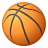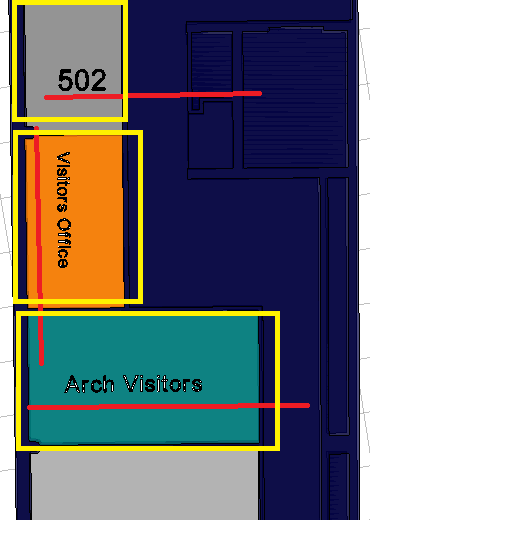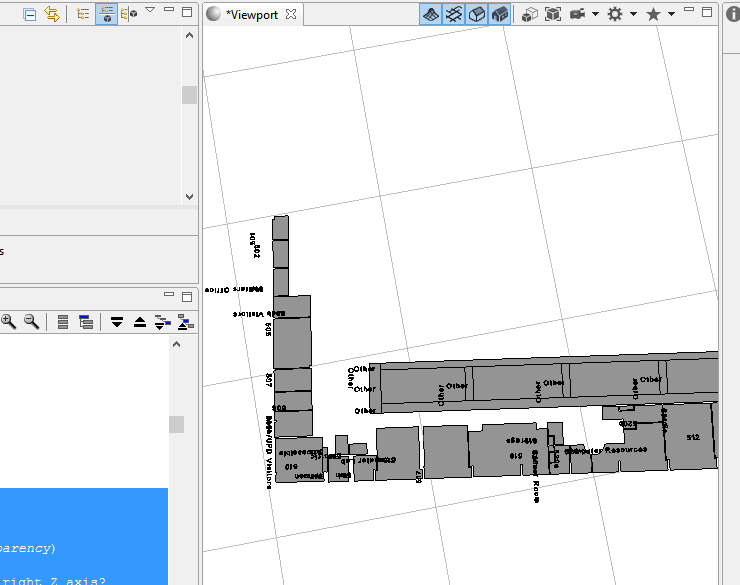# CityEngine Text Attribute Rule

3541
4
06-29-2015 09:21 PMby
New Contributor III

Hi,

I would like to use a Rule to create a text from attributes that would be embedded into a room polygon, and base on its length (horizontal or vertical) (See the attachment) also wit would be good to scale text base on the polygon size

This example I use one Rule per Room - that's why its different orientation/ sizeAnd here is the Rule I have been using,

Can you help me modify this Rule, so I can use only one Rule for all my floors ( and get all the room names - scaled and placed horizontal/vertical- based on it room area?)

Thank you!

Lukasz

/**

* File:    3D_Text.cga

* Created: 13 May 2014

* Changed: 19 Aug 2014

* Author:  chri7180

*/

version "2014.0"

# Important Note: Procedural Runtime setting for Max Derivation Depth

# must be raised above default for rule to print longer text.

# Go to Edit > Preferences > General > Procedural Runtime.

# Set Max Derivation Depth to 1000 (an arbitrarily large value).

# USEFUL FOR TESTING:

#    the quick brown fox jumps over the lazy dog

#    THE QUICK BROWN FOX JUMPS OVER THE LAZY DOG

#    ABCDEFGHIJKLMNOPQRSTUVWXYZabcdefghijklmnopqrstuvwxyz0123456789,.'[]{}~!@#\$%^&()-_=+*:><|?/\

#    Special Char Test: ;,.'[]{}~!@#\$%^&()-_=+*:><|?/\

# To make more fonts, email cwilkins@esri.com for instructions.

# Change below directories for your use.

const assetsDirectory = "assets/3D_Text/" + Font + "/"

const assetsDirectoryLower = assetsDirectory + "Lower/"

@Group("DISPLAY", 10) @Order(10)

@Order(1)

attr Text = "Floating;3D TEXT;is Cool"

#attr Text = "ABCDEFGHIJKLMNOPQRSTUVWXYZabcdefghijklmnopqrstuvwxyz0123456789,.'[]{}~!@#\$%^&()-_=+*:><|?/"

@Group("DISPLAY") @Order(20)

@Color

attr Color = "#00FF00"

@Group("DISPLAY") @Order(30)

attr White_Underlay = false

@Group("DISPLAY") @Order(40)

@Range(0,1)

attr Transparency = 0

@Group("DISPLAY") @Order(50)

@Range("Arial")

attr Font = "Arial"

@Group("POSITION & SIZE", 20) @Order(10)

@Range(0,200)

attr Elevation = 0.2

@Group("POSITION & SIZE") @Order(20)

@Range(1,5)

attr Font_Height = 1

@Group("POSITION & SIZE") @Order(30)

@Range(0,100)

attr Font_Depth = 0.2

@Group("POSITION & SIZE") @Order(40)

@Range(-180,180)

attr Rotation_Y = 90

@Group("POSITION & SIZE") @Order(50)

@Range(-90,90)

attr Tilt = 90

@Group("RELATIVE SIZE", 30) @Order(60)

@Range(0,1)

attr Kerning = 0.15

@Group("RELATIVE SIZE") @Order(70)

@Range(0,1)

attr Stretch_Horizontal = 0

@Group("RELATIVE SIZE") @Order(80)

@Range(0,1)

attr Line_Spacing = 0.5

@Group("RELATIVE SIZE") @Order(90)

@Range(0,1)

attr Space_Size = 0.3

@StartRule

Generate -->

color(Color)

set(material.opacity, 1 - Transparency)

alignScopeToAxes(y)

# XX Is Tilt tilting around the right Z axis?

r(-Tilt, Rotation_Y, 0)

translate(rel, world, 3, Elevation, -1)

PrintLines(Text, 1)

@Hidden

attr Line = ""

@Hidden

attr ReturnAt = 0

const Max_Lines = 20

# FIXME: multiple semi-colons in a row just make spaces. Not sure why. Not important for now.

PrintLines(myString, lineIndex) -->

case len(myString) <= 0 || lineIndex > Max_Lines:

NIL

else:

case find(myString, ";", 2) > 0:

set(ReturnAt, find(myString, ";", 0))

PrintLetters(substring(myString, 0, ReturnAt))

t(0, -(Font_Height * (1 + Line_Spacing)), 0)

r(0,0,0)

t(0,0,0)

PrintLines(substring(myString, ReturnAt + 1, Max_String_Length), lineIndex + 1)

else:

PrintLetters(substring(myString, 0, Max_String_Length))

NIL

# Used during recursion on Text:

@Hidden

attr Letter = ""

@Hidden

attr Asset = ""

const Max_String_Length = 1000

PrintLetters(myString) -->

PrintLetters(myString, len(myString))

PrintLetters(myString, n) -->

case n > 0:

set(Letter, substring(myString, 0, 1))

set(Asset, getLetterAsset(Letter))

PrintLettersHandleCursorMoves(myString, n)

else:

NIL

PrintLettersHandleCursorMoves(myString, n) -->

case Letter == " ":

# Printing a space only moves the cursor. No asset used.

PrintLettersRecurse(myString, n)

else:

PrintLettersAssetSetup(myString, n)

PrintLettersAssetSetup(myString, n) -->

case !fileExists(Asset):

print("Error: No file for asset='"+Asset+"'")

PrintLettersRecurse(myString, n)

else:

# This one letter is printed in a separate branch.

PrintLetter

PrintLettersRecurse(myString, n)

PrintLettersRecurse(myString, n) -->

t((_assetSizeX  + Kerning + Stretch_Horizontal) * Font_Height, 0, 0)

PrintLetters(substring(myString, 1, Max_String_Length), n - 1)

_assetSizeX =

case Letter == " ":

Space_Size

else:

sx(Asset)

PrintLetter -->

s(0,0,0)

insert(Asset, Font_Height)

insert(a, scalar) -->

i(a)

s(scalar * sx(a), scalar * sy(a), scalar * sz(a))

t((scalar * tx(a)) - tx(a),(scalar * ty(a)) - ty(a),(scalar * tz(a)) - tz(a))

s('(1 + Stretch_Horizontal),'1,0)

WhiteUnderlay

sx(asset) = assetInfo(asset, sx)

sy(asset) = assetInfo(asset, sy)

sz(asset) = assetInfo(asset, sz)

tx(asset) = assetInfo(asset, tx)

ty(asset) = assetInfo(asset, ty)

tz(asset) = assetInfo(asset, tz)

WhiteUnderlay -->

case White_Underlay:

# Weird push/pop notation that I've never seen anyone use:

[

color(1,1,1)

extrude(underlayDepth)

X.

]

t(0, 0, underlayDepth)

extrude(overlayDepth)

X.

else:

extrude(Font_Depth_Non_Zero)

const flatUnderlayPercent = 0.05

const underlayDepth = Font_Depth_Non_Zero * (1 - flatUnderlayPercent)

const overlayDepth = Font_Depth_Non_Zero * (flatUnderlayPercent)

const Font_Depth_Non_Zero =

case Font_Depth <= 0:

Font_Height * 0.05

else: Font_Depth

#######################################

# Letter Assets - this finds the OBJ file for each letter.

getLetterAsset(letter) =

# These characters cannot be used for filenames:  *!:><|?/\

# Below case structure relates those characters to specially named assets.

# The else case is for all other characters.

case letter == " " : ""

case letter == "*" : getAssetPath(letter) + "_asterisk.obj"

case letter == "!" : getAssetPath(letter) + "_bang.obj"

case letter == ":" : getAssetPath(letter) + "_colon.obj"

case letter == ">" : getAssetPath(letter) + "_greaterthan.obj"

case letter == "<" : getAssetPath(letter) + "_lessthan.obj"

case letter == "|" : getAssetPath(letter) + "_pipe.obj"

case letter == "?" : getAssetPath(letter) + "_question.obj"

case letter == "/" : getAssetPath(letter) + "_slash.obj"

case letter == "\\" : getAssetPath(letter) + "_backslash.obj"

case letter == "\"" : getAssetPath(letter) + "_quote.obj"

else: getAssetPath(letter) + letter + ".obj"

getAssetPath(letter) =

case isLowercase(letter): assetsDirectoryLower

else: assetsDirectory

isLowercase(letter) =

case letter == "a" : true

case letter == "b" : true

case letter == "c" : true

case letter == "d" : true

case letter == "e" : true

case letter == "f" : true

case letter == "g" : true

case letter == "h" : true

case letter == "i" : true

case letter == "j" : true

case letter == "k" : true

case letter == "l" : true

case letter == "m" : true

case letter == "n" : true

case letter == "o" : true

case letter == "p" : true

case letter == "q" : true

case letter == "r" : true

case letter == "s" : true

case letter == "t" : true

case letter == "u" : true

case letter == "v" : true

case letter == "w" : true

case letter == "x" : true

case letter == "y" : true

case letter == "z" : true

else: false

4 Repliesby Anonymous User
Not applicable

You would need to import the 3D text rule into your room rule, then try different ways of scaling a start shape for the text rule, probably deriving it from the floor of the room. There are numerous attributes to control the text size and whatnot, and you would want to set those based on the length of the string to display (use len() CGA function for that). I haven't used the rule in a while, so I don't know it off the top of my head.

Note: The rule uses OBJ models for each letter, and are very heavy in poly count. (might slow down your scene)

Chrisby
New Contributor III

Thank you Chris,

Do you know if CItyEngine 2015 supports labels?

Lukaszby Anonymous User
Not applicable

It does not support labels.by
New Contributor III

Hi Chris

Can you help me modify the Rule, so the labels are horizontal and in the center of each room?

I think it is your Rule (3d text)

Generate -->

color(Color)

set(material.opacity, 1 - Transparency)

alignScopeToAxes(y)

# XX Is Tilt tilting around the right Z axis?

r(-Tilt, Rotation_Y, 0)

center(xy)

translate(rel, world, 0, Elevation, -4)